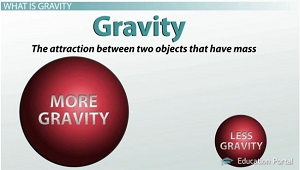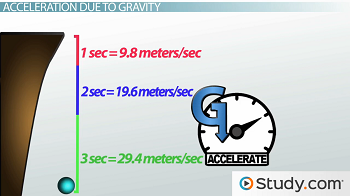# Newton's Laws and Weight, Mass & Gravity

An error occurred trying to load this video.

Try refreshing the page, or contact customer support.

Coming up next: Classical Relativity: Distance and Time Relation to the Observer

### You're on a roll. Keep up the good work!

Replay
Your next lesson will play in 10 seconds
• 0:03 Newton's Laws and…
• 1:09 What is Gravity?
• 2:39 Acceleration Due to Gravity
• 4:10 Gravity and Weight
• 4:46 Calculation of Weight
• 5:47 Big Misconception

Want to watch this again later?

Timeline
Autoplay
Autoplay
Speed

#### Recommended Lessons and Courses for You

Lesson Transcript
Instructor: Jim Heald

Jim has taught undergraduate engineering courses and has a master's degree in mechanical engineering.

Did you know that mass and weight are not the same? This lesson describes the difference between the two as well as the effect of gravity on weight. Examples are used to teach you how to calculate weight based on mass and acceleration of gravity.

## Newton's Laws: Weight, Mass and Gravity

Most of us have seen images of men walking on the moon. Now, even though the astronauts are wearing really heavy suits, they seem to bounce around the surface of the moon with very little effort. How is it that we can bounce around on the moon with ease while jumping here on Earth requires a lot of effort? The answer to this question lies within the difference between mass and weight.

Mass is a measure of how much matter an object contains, while weight is a measure of the force of gravity on the object. An object has the same composition, and therefore mass, regardless of its location. For example, a person with a mass of 70 kg on Earth has a mass of 70 kg in space as well as on the moon. However, that same person's weight is not the same since gravity is different in these locations. The person will weigh less on the moon because the moon has less gravity. To better understand the concepts of weight and mass, we must first consider gravity and its effect on objects.

## What is Gravity?

So what is gravity? Gravity is the attractive pull between two objects that have mass. The strength of gravity is directly proportional to the amount of mass of each object. In other words, the larger the objects, the greater the gravitational attraction between them. For example, the gravitational pull you experience on Earth is much greater than it would be on the moon because the Earth's mass is greater. An object with twice as much mass will exert twice as much gravitational pull on other objects.On the other hand, the strength of gravity is inversely related to the square of the distance between two objects. For example, if the distance between two objects doubles, meaning they're twice as far apart, the gravitational pull decreases by a factor of 4. This is because 2 squared is equal to 4. This means the effect of distance on gravitational attraction is greater than the effect of the masses of the objects.

## Gravity as a Force

Gravity is a force. A force is simply a push or a pull experienced by objects that interact with each other. The interaction can be direct or at a distance, which is the case of gravity. Newton's laws tell us that if an unbalanced force acts on an object, it will change the object's state of motion. In other words, the object will accelerate. Since gravity is a force, gravity causes objects to accelerate.

## Acceleration Due to Gravity

Let's look at an example of how gravity causes acceleration. If you drop a ball from a cliff, you will notice that the speed increases as it falls - it accelerates due to gravity. We have determined the acceleration of gravity is 9.8 m/sec^2 - that is, for free-falling objects on Earth. Free falling simply means no other forces, except gravity, are acting on the object. For example, any effect of wind resistance would be neglected. The velocity of a free-falling object increases by 9.8 meters per second every second.

Let's look at the speed of the ball as it drops over time. This is going to help us understand how gravity causes acceleration.As you see on the screen, the ball will accelerate to a speed of 9.8 meters per second in the first second of travel. Over the next second, the speed of the ball will again increase by 9.8 meters per second, meaning it's traveling at 19.6 meters per second. The same thing will happen during the third second of time, so the ball will be traveling at 29.4 meters per second. With each second, the ball's speed increases by 9.8 meters per second.

The acceleration of gravity is so important that it has its own symbol. It is often abbreviated with the letter g. g = 9.8 m/sec^2 - that's the acceleration of gravity here on Earth for free-falling objects.

## Gravity and Weight

Well, what about weight? Weight is a measure of the force of gravity acting on an object. According to Newton's laws of motion, force is directly proportional to both mass and acceleration, and the equation for force is F = m * a, where m = mass and a = acceleration. We can use this equation to solve for weight. All objects on Earth, whether they are falling, thrown, or even sitting still, experience the effect of gravity. Therefore, we can determine the weight of an object using the acceleration of gravity.

To unlock this lesson you must be a Study.com Member.

### Register to view this lesson

Are you a student or a teacher?

### Unlock Your Education

#### See for yourself why 30 million people use Study.com

##### Become a Study.com member and start learning now.
Back
What teachers are saying about Study.com

### Earning College Credit

Did you know… We have over 160 college courses that prepare you to earn credit by exam that is accepted by over 1,500 colleges and universities. You can test out of the first two years of college and save thousands off your degree. Anyone can earn credit-by-exam regardless of age or education level.